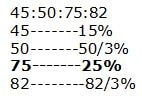# gkrecall

We brings you daily Current affairs, daily Current Affairs Quiz, weekly Current Affairs, weekly Current Affairs Quiz to enhance your preparation for upcoming exams. We also provide articles related to quant, reasoning, English, ssc etc .

# Arithmetic sum for RRB PO/Clerk mains

(1-5) Four neighbours Aakash, Billu, Chandan, and Deepak started a business together with initial investments in the ratio 2:4:5:6 respectively. At the end of one year, Billu withdrew Rs. 2500, Deepak withdrew Rs. 4000 rupees and the remaining two kept their investment unchanged. At the end of 2nd year, Deepak and Aakash invested some additional amounts and remaining two kept their investment unchanged such that the ratio of their investments becomes 5:3:5:6 respectively at the end of 2nd year. At the end of the third year, they share profit in the ratio of their investment and time of investment.

The profit of Aakash is what percent of the profit of Billu at the end of the third year?

1.   80%

2.   90%

3.   110%

4.   70%

5.   85%

2. Find the ratio of their profits at the end of 3 years?

1.   45:50:77:82

2.   45:50:72:81

3.   45:50:75:83

4.   45:50:75:82

5.   40:50:72:82

3. Find the difference between the additional amount invested by Aakash and Deepak at the end of the 2nd year?

1.   Rs. 3750

2.   Rs. 3200

3.   Rs. 3500

4.   Rs. 4200

5.   Rs. 3650

4. If Chandan will be given 16% of the profit for handling business and at the end of the third year if he receives a total Rs. 12300 as profit, then find total profit earned at the end of the third year?

1.   25000

2.   31000

3.   32000

4.   35000

5.   35000

5. Find the total investment done initially?

1.   Rs. 47500

2.   Rs. 42500

3.   Rs. 41250

4.   Rs. 45500

5.   Rs. 48000

6. In a school there are 950 students, if 60% boys know swimming but total 38% of the students don’t know swimming. Find how many girls know swimming. If number of boys are 230 more than the number of girls.

1.   245

2.   236

3.   235

4.   265

5.   225

7. In a department the no. of males and no. of females are in the ratio of 3:2. If the no. of males are increased by 10% and no. of females are increased by 19, then the new ratio of the no. of males to females is 4:3. What is the total strength of the department after increase?

1.   221

2.   245

3.   231

4.   233

5.   261

8. Total cost of three gadgets A, B and C is Rs. 7500 and ratio of cost of A to B is 2:3 while the cost of C is Rs. 1100 more than the cost of B. If A is sold at 20% profit and B is sold at 25% loss. At what price C should be sold for overall profit of 20%.

1.   Rs. 5230

2.   Rs. 5360

3.   Rs. 5480

4.   Rs. 5470

5.   Rs. 5280

9. Boat “A” which sails at 9km/hr in still water starts chasing Boat “B”, from 8km behind, another one which sails at 5km/hr in the upstream direction. After how long will it catch up if the stream is flowing at 2km/hr?

1.   1hr

2.   2hr

3.   3hr

4.   4hr

5.   5hr

10. To reach city B from city A, at 6am, Parag will have to travel at an average speed of 36km/hr. He will reach City B at 5am if he travels at 48km/hr. At what average speed should Parag travel to reach City B at 4am?

1.   60km/hr

2.   72km/hr

3.   84km/hr

4.   96km/hr

5.   108km/hr

Solution -

Explanation :

Aakash’s profit = 6x+y = 6*2500+7500 = 22500

Billu’s profit = 12x-5000 = 12*2500-5000 = 25000

Required percentage = 22500/25000 =( 9/10)*100 = 90%

Explanation:

Aakash’s profit = 6x+y = 6*2500+7500 = 22500

Billu’s profit = 12x-5000 = 12*2500-5000 = 25000

Chandan’s profit = 15x = 37500

Deepak’s profit = 18x-8000+z = 18*2500-8000+4000 = 41000

Ratio of profits = 225:250:375:410 = 45:50:75:82

Explanation:
y = 7500
z = 4000
Difference = 7500 – 4000 = 3500

Explanation:
Chandan is given 16% for handling
Profit left for all four for their business = 84%Chandan’s profit = 16%+25% = 12300
41% = 12300
1% = 300
100% = 30000

Explanation:
2x+4x+5x+6x = 17x = 17*2500 = 42500

Explanation –
Total boys = 230 + total girls
Total boys + total girls = 950
230 + total girls + Total girls = 950
2 × Total girls = 720
Total girls = 360
Total boys = 590
Total student who know swimming = 62% of 950 = 589
Total boys who know swimming = 60% of 590 = 354
Total girls who know swimming = 589-354 = 235

Explanation
Let the no. males initially are 3x
After increase 3.3x
No. of females initially = 2x
After increase = 2x + 19
According to question,
3.3x / 2x + 19 = 4 / 3
x = 40
Strength = 3.3x + 2x + 19 = 5.3x + 19 = 5.3 × 40 +19 = 231

8. Incorrect
Explanation-
Let the cost price of A = 2x
Cost price of B = 3x
Cost price of C 3x + 1100
Total cost = 2x + 3x + 3x + 1100 = 8x+1100
8x +1100 = 7500
x = 800
Cost of A = 1600
Cost of B = 2400
Cost of C = 3500
Profit on selling A = 20% of 1600 = 320
Loss on selling B = 25% 0f 2400 = 600
Required overall profit = 20% of 7500 = 1500
A + B + C = 1500
320 + (-600) + C = 1500
C = 1780
Hence, C should be sold at 3500 + 1780 = 5280

Explanation
Speed of boat A in upstream = 9-2=7
Relative speed of boat A with respect to boat B (in Upstream) = 7-5 = 2km/hr
Distance to cover = 8km/hr
Time taken = 8km/ relative speed = 8/2 = 4hr